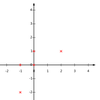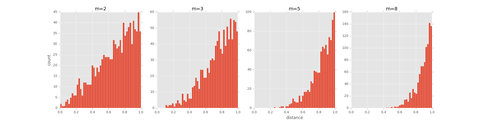PCA （Principal Component Analysis，主成分分析）

PCA通过正交变换将一组可能存在相关性的变量转换为一组线性不相关的变量，即把多指标转化为少数几个综合指标，转换后的这组变量就叫做主成分，其中每个主成分都能够反映原始变量的大部分信息，且所含信息互不重复，以此达到降维的目的。

1.算法步骤

1. 将原始数据按列组成列矩阵2.的每一行（代表一个属性字段）进行零均值化，即减去这一行的均值；
3. 求出协方差矩阵4. 求出协方差矩阵的特征值及对应的特征向量；
5. 将特征向量按对应特征值大小从上到下按行排列成矩阵，取前行组成矩阵6.即为降维到k维后的数据。

2.数学原理

PCA中有两个很重要的思想：

• 最大可分性思想，即样本点在超平面上的投影能尽可能的分开。基于最大可分性思想，我们要找的方向是使得降维后损失最小的方向，可以理解为投影后的数据尽可能的分开，这种分散程度可以用数学上的方差来表示，方差越大数据越分散。
• 主成分相互独立思想，即主成分之间不能存在线性相关性的，不然的话必然存在重复表示的信息，那么第二个主成分只能在与第一个主成分正交的方向上选择。t-SNE

t-SNE算法仿射(affinitie)变换将数据点映射到概率分布上，利用条件概率来衡量数据点之间的相似性，通过使数据集在高维和低维两个空间的条件概率尽可能接近，将数据从高维空间映射到低维空间。通过t-SNE降维不仅可以保持数据的差异性，而且可以很好地保持数据的局部结构。

1.算法步骤

t-SNE算法步骤如下图所示。

2.数学原理

（0）算法的输入有两个：

1.维数据；
2.，困惑度。困惑度可以解释为一个点附近的有效近邻点个数，SNE对困惑度的调整比较有鲁棒性，通常选择5-50之间。

（1）是SNE算法中设置的一个条件概率用来衡量高维数据的相似性，衡量的是数据点作为数据点的邻域的概率；是方差，这个方差大小与输入的困惑度有关。

（2）是为了解决引入异常值的问题，比如是异常值，那么会很大，对应的所有的都会很小，导致低维映射下的对损失函数影响很小。为了解决这个问题，将联合概率分布定义修正为：， 使得每个点对于损失函数都会有一定的贡献。

（3）—（4）不太理解为什么维的，按道理说是最终的输出，维度应该是（5）是SNE算法中设置的一个条件概率用来衡量低维数据的相似性。

（6）表示低维数据集中所有其他数据点之间的相对条件概率。

（7）是目标函数(cost function)，是两个分布之间的距离-KL散度(Kullback-Leibler divergences)。

（4）（5）（6）（7）是个循环，通过不断地利用梯度下降算法，得到最优解。

Auto Encoders（自编码器）

"""
To know more or get code samples, please visit my website:
https://morvanzhou.github.io/tutorials/
Or search: 莫烦Python
Thank you for supporting!
"""

# please note, all tutorial code are running under python3.5.
# If you use the version like python2.7, please modify the code accordingly

# 9 - Autoencoder example

# to try tensorflow, un-comment following two lines
# import os
# os.environ['KERAS_BACKEND']='tensorflow'
import numpy as np
np.random.seed(1337)  # for reproducibility

from keras.datasets import mnist
from keras.models import Model
from keras.layers import Dense, Input
import matplotlib.pyplot as plt

# download the mnist to the path '~/.keras/datasets/' if it is the first time to be called
# X shape (60,000 28x28), y shape (10,000, )
(x_train, _), (x_test, y_test) = mnist.load_data()

# data pre-processing
x_train = x_train.astype('float32') / 255. - 0.5       # minmax_normalized
x_test = x_test.astype('float32') / 255. - 0.5         # minmax_normalized
x_train = x_train.reshape((x_train.shape, -1))
x_test = x_test.reshape((x_test.shape, -1))
print(x_train.shape)
print(x_test.shape)

# in order to plot in a 2D figure
encoding_dim = 2

# this is our input placeholder
input_img = Input(shape=(784,))

# encoder layers
encoded = Dense(128, activation='relu')(input_img)
encoded = Dense(64, activation='relu')(encoded)
encoded = Dense(10, activation='relu')(encoded)
encoder_output = Dense(encoding_dim)(encoded)

# decoder layers
decoded = Dense(10, activation='relu')(encoder_output)
decoded = Dense(64, activation='relu')(decoded)
decoded = Dense(128, activation='relu')(decoded)
decoded = Dense(784, activation='tanh')(decoded)

# construct the autoencoder model
autoencoder = Model(input=input_img, output=decoded)

# construct the encoder model for plotting
encoder = Model(input=input_img, output=encoder_output)

# compile autoencoder

# training
autoencoder.fit(x_train, x_train,
epochs=20,
batch_size=256,
shuffle=True)

# plotting
encoded_imgs = encoder.predict(x_test)
plt.scatter(encoded_imgs[:, 0], encoded_imgs[:, 1], c=y_test)
plt.colorbar()
plt.show()

PCA 的数学原理和可视化效果www.jianshu.com机器之心：基于TensorFlow理解三大降维技术：PCA、t-SNE 和自编码器zhuanlan.zhihu.comt-SNE完整笔记www.datakit.cn机器学习: t-Stochastic Neighbor Embedding 降维算法 (一)blog.csdn.net